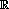Vectors and conics

This free course is available to start right now. Review the full course description and key learning outcomes and create an account and enrol if you want a free statement of participation.

Free course

Vectors and conics

Introduction

The idea of vectors and conics may be new to you. In this course we look at some of the ways that we represent points, lines and planes in mathematics.

In Section 1 we revise coordinate geometry in two-dimensional Euclidean space,2, and then extend these ideas to three-dimensional Euclidean space,3. We discuss the equation of a plane in3, but find that we do not have the tools to determine the equation of a plane, and leave this until Section 3.

In Section 2 we introduce the idea of a vector, and look at the algebra of vectors. Vectors give us a way of looking at points and lines, in the plane and in3, which is sometimes more useful than Cartesian coordinates, although the two are closely related.

In Section 3 we introduce the idea of the dot product of two vectors, and then use it to determine the general form of the equation of a plane in3.

In Section 4 we explain the origin of conics, as the curves of intersection of double cones and planes in3. The focus–directrix definitions of the non-degenerate conics, the ellipse, the parabola and the hyperbola, are given. We observe that conics are precisely the subsets of the plane determined by an equation of degree two.

This OpenLearn course is an adapted extract from an Open University course M208 Pure Mathematics [Tip: hold Ctrl and click a link to open it in a new tab. (Hide tip)]

M208_1